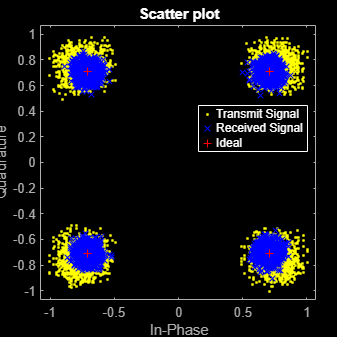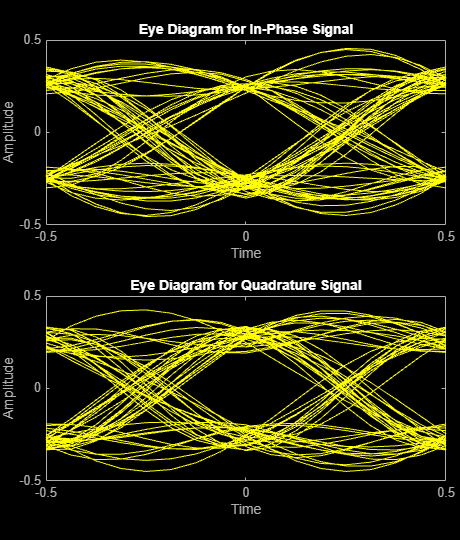# Scatter Plot and Eye Diagram with MATLAB Functions

This example shows how to visualize signal behavior through the use of eye diagrams and scatter plots. The example uses a QPSK signal which is passed through a square-root raised cosine (RRC) filter.

### Scatter Plot

Set the RRC filter, modulation scheme, and plotting parameters.

```span = 10; % Filter span rolloff = 0.2; % Rolloff factor sps = 8; % Samples per symbol M = 4; % Modulation alphabet size k = log2(M); % Bits/symbol phOffset = pi/4; % Phase offset (radians) n = 1; % Plot every nth value of the signal offset = 0; % Plot every nth value of the signal, starting from offset+1```

Create the filter coefficients using the `rcosdesign` function.

`filtCoeff = rcosdesign(rolloff,span,sps);`

Generate random symbols for an alphabet size of `M`.

```rng default data = randi([0 M-1],5000,1);```

Apply QPSK modulation.

`dataMod = pskmod(data,M,phOffset);`

Filter the modulated data.

`txSig = upfirdn(dataMod,filtCoeff,sps);`

Calculate the SNR for an oversampled QPSK signal.

```EbNo = 20; snr = EbNo + 10*log10(k) - 10*log10(sps);```

Add AWGN to the transmitted signal.

`rxSig = awgn(txSig,snr,'measured');`

`rxSigFilt = upfirdn(rxSig, filtCoeff,1,sps);`

Demodulate the filtered signal.

`dataOut = pskdemod(rxSigFilt,M,phOffset,'gray');`

Use the `scatterplot` function to show scatter plots of the signal before and after filtering. You can see that the receive filter improves performance as the constellation more closely matches the ideal values. The first `span` symbols and the last `span` symbols represent the cumulative delay of the two filtering operations and are removed from the two filtered signals before generating the scatter plots.

```h = scatterplot(sqrt(sps)*txSig(sps*span+1:end-sps*span),sps,offset); hold on scatterplot(rxSigFilt(span+1:end-span),n,offset,'bx',h) scatterplot(dataMod,n,offset,'r+',h) legend('Transmit Signal','Received Signal','Ideal','location','best')```### Eye Diagram

Display 1000 points of the transmitted signal eye diagram over two symbol periods.

`eyediagram(txSig(sps*span+1:sps*span+1000),2*sps)`Display 1000 points of the received signal eye diagram.

`eyediagram(rxSig(sps*span+1:sps*span+1000),2*sps)`Observe that the received eye diagram begins to close due to the presence of AWGN. Moreover, the filter has finite length which also contributes to the non-ideal behavior.